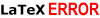# latex rendering server\red\large e^x=\sum_{n=0}^\infty\frac{x^n}{n!}

\blue\normal\left {\begin{x+\frac{3x-y}{x^2+y^2}=3}\\{y-\frac{x+3y}{x^2+y^2}=0}

\normal f^\prime(x)\ = \lim_{\Delta x\to0}\frac{f(x+\Delta x)-f(x)}{\Delta x}

R=\normal \frac{\displaystyle{\sum_{i=1}^n (x_i-\bar{x})(y_i- \bar{y})}}{\displaystyle{\left[ \sum_{i=1}^n(x_i-\bar{x})^2 \right]^{1/2}}}

\large \sum_{i=1}^{n}\frac{aibi}{ai+bi} \leq \frac{(\sum_{i=1}^{n}ai)(\sum_{i=1}^{n}bi)}{\sum_{i=1}^{n}ai+\sum_{i=1}^{n}bi}

Some example expressions rendered by MimeTeX (it’s good to appear to be smarter than you are 😬!) If an expression fails to be rendered, you would see an error image like this:ecently, the wonderful yourequations.com site (which I’ve been using to occasionally render mathematical expressions on web pages) has ceased it’s service due to heavy traffic. I was thinking about running my own LaTeX rendering server, things turned out to be pretty easy as follow, thanks to the excellent MimeTeX package, a LaTeX reduced subset. It’s also interesting to experiment the “stone age technique” of CGI, first download and compile the package:

wget http://www.forkosh.com/mimetex.zip
unzip mimetex.zip
cd mimetex
cc -DAA mimetex.c gifsave.c -lm -o latex.cgi
# test the binary, view the ‘fermat.gif’ image
./latex.cgi -i “a^2+b^2=c^2” -e fermat.gif

Uploaded to host, latex.cgi runs without any dependencies. The ugly thing with my (free) Linux host is that although it does allow CGI, it doesn’t allow CGI to return documents of type ‘image/gif’ no matter what. To work around, I wrote a small PHP script, which parses the GET input, calls CGI to generate and save image in a cache directory, then redirects request to the LaTeX image. This also helps not to expose your CGI directly on the web too!

// use ‘system’ command to execute CGI
$cmd = “$mimetex_path -e “.$full_filename;$cmd = $cmd.” “.escapeshellarg($formula);
system($cmd,$status_code);
$text =$pictures_path.”/”.$filename; return$text;

I’ve been always loving CGI for its simplicity, CGI, Perl, Python… old things never die! Although I have almost no experiences with them, they let you do whatever you want to given a little tweaking know – hows. You can go and test my LaTeX rendering server at http://tkxuyen.com/latex.php, the url syntax: latex.php?formula=$$latex code$$, here is an example. Please note that MimeTeX is not as full – featured as LaTeX, it can’t render some too – complex expressions and it uses an ugly bitmap font. If your server has some LaTeX support, consider using MathTeX, a more advanced version from the same author.